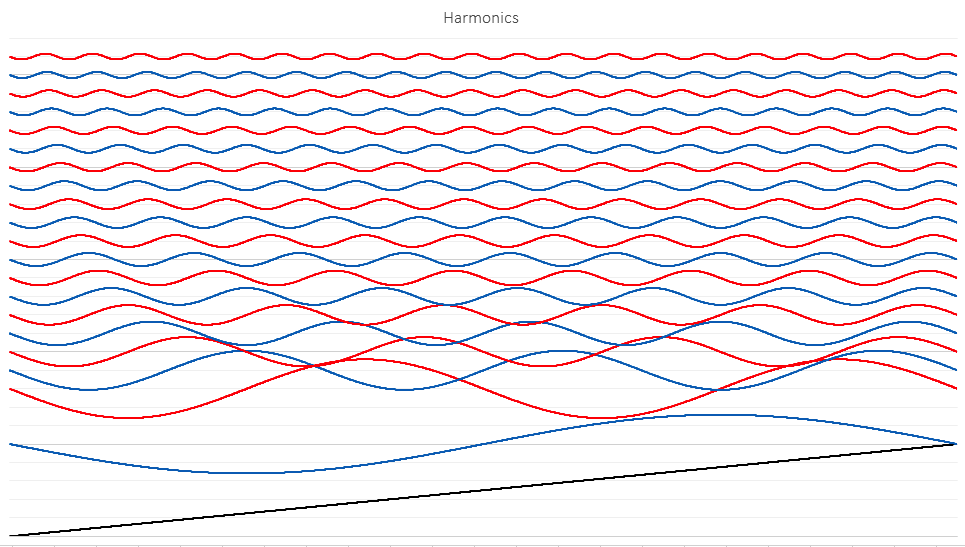# Simple math

Published on April 6, 2019

I issued a challenge to my colleagues and no one would come up with the answer. I even offered a free copy of my book on harmonics, Distortion, but the problem is, nobody can understand the answer until they read Chapter 2 of my book, so it's a Kobayashi Maru, a no-win scenario. Too bad, I love thrusting my book into fresh faces, waiting for their opinion and hoping for a review. On Amazon, reviews make the product.

So in this challenge I asked this question: If I take the spectrum of a Ramp wave and remove all the Odd (blue) harmonics, I get two ramps at half amplitude. Why does that happen? The answer is super easy, it's just simple math, and yet it's so cool it will flummox a Vegan Snow Lizard. Here is how it works: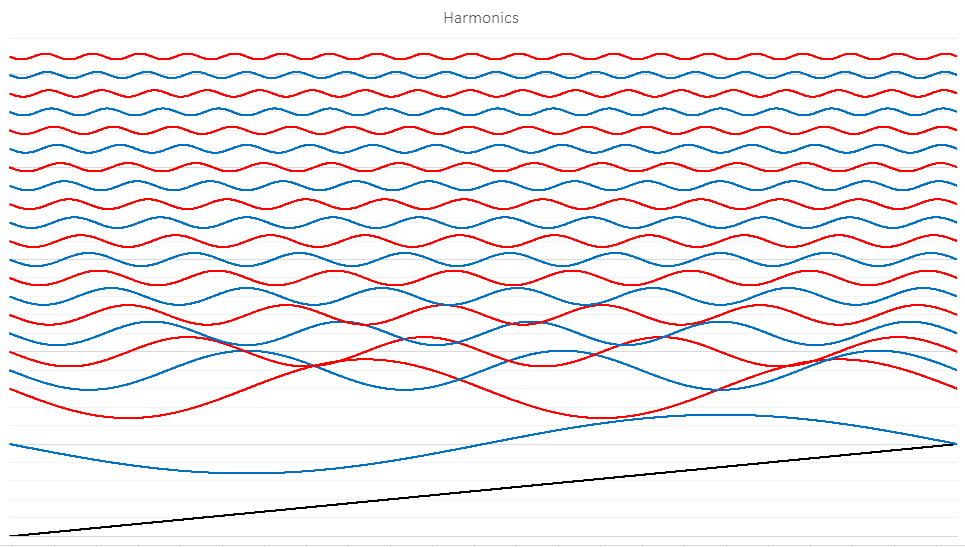Above I show the first 20 harmonic constituents of a simple ramp wave that goes from -1V to +1V, and then instantly returns to -1V at the end. Given this wave, I get the fundamental (the first blue wave at the bottom of the image) and then harmonics, both Odd and Even that fall off at the rate of 1/harmonic#, just like in a square wave. (In chapter 2 of the book this behavior heralds an important milestone, a light should turn on in your head, but we don't have time for that here.) Note that in all these pictures, I scaled harmonics 2-20 up by a factor of 2 to make it easier to see them, especially the smaller ones. The only difference between a square wave and a ramp wave in fact is that a square wave contains no Even harmonics, so if I remove the Even harmonics from the wave above, I get this: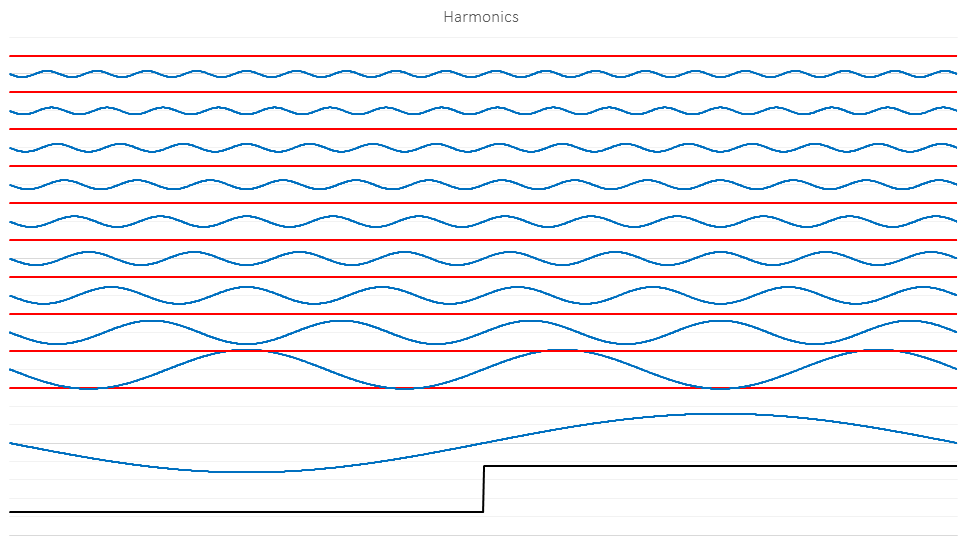Now that the Even harmonics are gone, the Odd harmonics conspire to square up the fundamental, turning it from a sine wave into a square wave, as long as the Odd harmonics fall off at 1/harmonic#. Note that the square wave is now one half the amplitude of the original ramp wave. You will see that play out in a minute.

OK, so we can see how that works, but if I remove the Odd harmonics instead, I get this: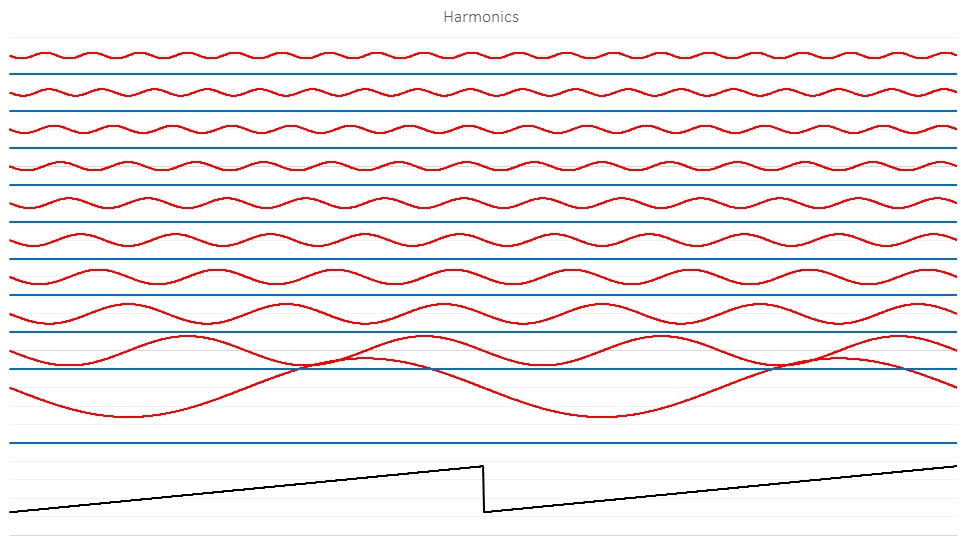So now the question is, why? The reason is really simple, you're going to hate yourself for not getting it. The "fundamental" is no longer the fundamental anymore, since it's been zeroed out. The new fundamental is the former 2nd harmonic, making the former 4th harmonic the 2nd harmonic, and making the former 6th harmonic the 3rd harmonic. How does that play out?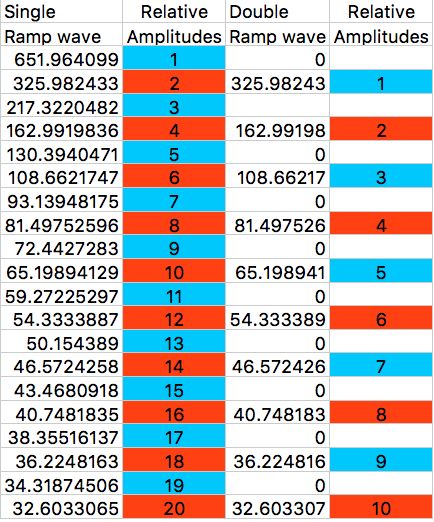This table shows how the amplitudes of the harmonics fall off. In the Single ramp wave, the fundamental has an amplitude of 641.96... let's call it 642. That's what the Excel FFT gave us. It's scaled oddly, which happens with every FFT you use, so let's just ignore the value and say that since that is the fundamental, that is a value of 1 (642/642). Now in the Single wave column you can see how the amplitudes go down by the amplitude number, the second harmonic is 1/2, the third harmonic is 1/3 and so on. For example, 642/326 = 2, the second harmonic is half the amplitude of the fundamental, while the third harmonic is 642/217.32 = 3, so the third harmonic is one third the amplitude of the fundamental. The fourth harmonic is 642/163 = 4 meaning that the fourth harmonic is one fourth the amplitude of the fundamental, and so on.

Now, if we remove the Odd harmonics from the Single wave spectrum and do an Inverse FFT, what happens? The old fundamental, harmonic #1 is gone, so harmonic #2 must take its place, which has an amplitude of 326. So that bin now has a gain of unity. The former 3rd harmonic is zero, since it was Odd, but the former 4th harmonic which has an amplitude of 163 is now one half the amplitude of the new fundamental, and 326/163=2, so that means that the new second harmonic has a relative amplitude of 1/2 or one half the amplitude of the fundamental. The new third harmonic (which was the original 6th harmonic) is 108.66, and 326/108.66=3, so the third harmonic is one third the amplitude of the fundamental. And what kind of wave has all (Odd and Even) harmonics falling off at 1/harmonic#? That's right, a Ramp wave, but since the second harmonic happens twice in this one cycle, there are two cycles, and since the new fundamental, the old second harmonic had half the amplitude of the original fundamental (second harmonic = 1/half the amplitude of the fundamental) then the entire wave overall has half the amplitude of the original ramp wave!

It seems crazy that a sequence of 1, 0.5, 0.33333, 0.25, 0.2, 0.16666... would have the same sequence with the Odd harmonics removed, but that's the way it works. It's just simple math, and that's the truth!